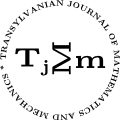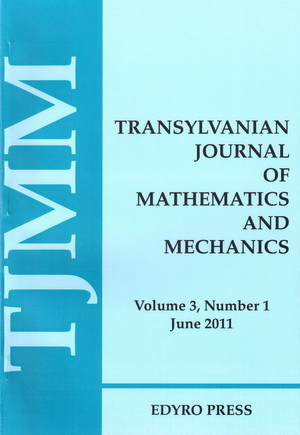ISSN: 2067-239X
ISSN(on-line): 2067-239X

Indexed in:
Mathematical Reviews
Zentralblatt MATH
EBSCO

Front cover# Volume 2 (2010), Number 2

## On the Al-Salam and Verma orthogonal q-polynomials

### Author(s): A. BOUANANI, L. KHÉRIJI, AND M. IHSEN. TOUNSI

Abstract: The aim of this paper is to highlight a new integral representation of the Al-Salam and Verma regular form through a true function by investigating the quadratic operator $\sigma$, duality and the ${H}_{q}$ -classical character of the Wall form where ${H}_{q}$ is the q-difference operator. Some other characterizations are given in the general case and in the symmetric one corresponding to the Al-Salam and Verma polynomials.

## Quaternion interval numbers and a matrix approach to them

### Author(s): CENNET BOLAT AND AHMET İPEK

Abstract: This paper is an extension of the work [Quaternions: further contributions to a matrix oriented approach, Linear Alg. and its Appl., 326 (2001), 205,-213.] , in which the representation matrix of a quaternion with real number coefficients is explicitly described, and then investigated properties of the fundamental real matrix associated with a quaternion. In this new paper, we extend the powerful ideas in that study to the quaternions with real interval coefficients. Many number of concepts and techniques that we learned in a standard setting for quaternions with real number coefficients, real intervals and matrices can be carried over to quaternion interval numbers.

## On a Sharp Inequality for the Medians of a Triangle

### Author(s): JIAN LIU

Abstract: In this paper, we prove that the known inequality which involving the upper bounds of median sums for the triangle is sharp. We also prove a stronger conjecture for this inequality, which is equivalent to a inequality posed by H.Y.Yin in . Finally, a similar conjecture checked by the computer is put forward.

## A Note on the Numerical Range of a Complex Tridiagonal Matrix

### Author(s): FATMA FEYZA TOPAL AND AHMET İPEK

Abstract: This paper is an extension of the work [Numerical range of a continuant matrix, Appl.Math.Lett., 14 (2001) 213-216.], in which the numerical range of a continuant matrix is explicitly described as an elliptic disc. In this new paper, we improve the results of that work.

## On Newton's Quadrature Formula for Mappings of Bounded Variation

### Author(s): MARIUS NICOLAE HELJIU

Abstract: An estimation of remainder for Newton`s quadrature formula for mappings of bounded variation are given. Also, some applications to special means are given.

## The Optimization of Simple Regression Models

### Author(s): CONSTANTIN ZĂVOIANU AND FELICIA ZĂVOIANU

Abstract: This paper contains an optimization algorithm for simple regression models, which has the purpose of selecting the best regression equation, if, for the same set of statistical data, several statistically valid models exist. Furthermore, the paper presents a method of determining the confidence intervals of the parameters of the non-linear simple regression models by operating, simultaneously, both with the original model as well as with the corresponding linearized model.